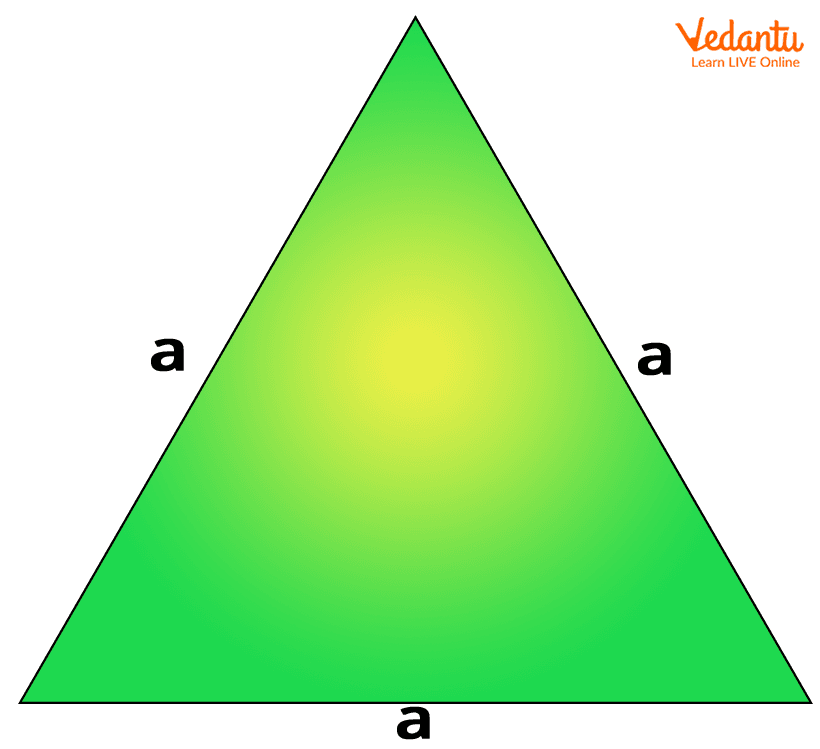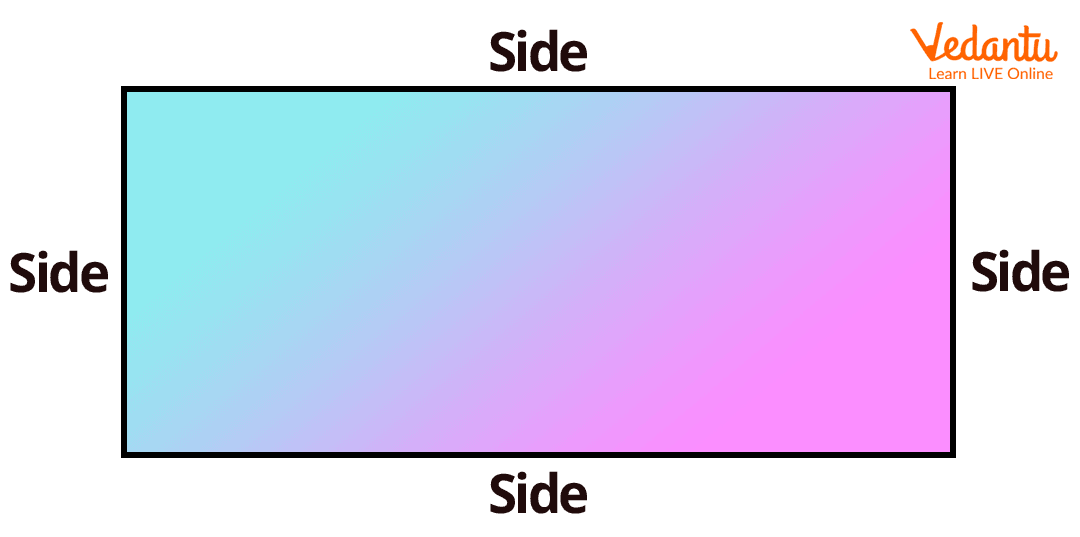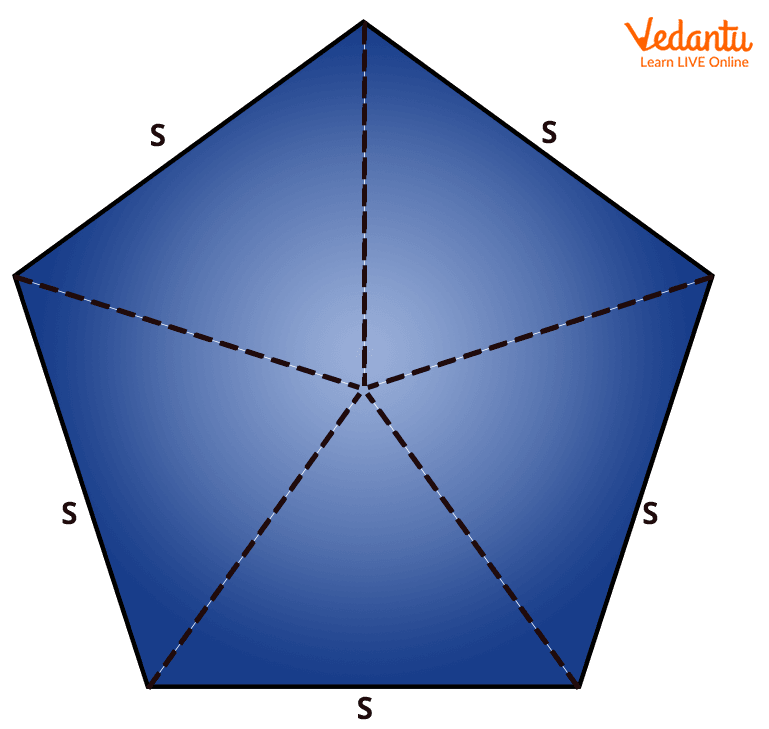Courses
Courses for Kids
Free study material
Offline Centres
More

# Side Definition in Maths – Meaning, Examples, and FAQsLast updated date: 29th Nov 2023
Total views: 158.7k
Views today: 3.58kThe concept of side in Maths is something very fundamental, and this concept should be picked up almost spontaneously by kids as they play around with toys or are curious about objects. The knowledge of side in Mathematics or in general is very basic and should be absorbed and retained for life. Let us learn more about side definitions in Maths in this article.

## Meaning of Side in Maths

In any two-dimensional figure, the line segment that joins two vertices is known as a side of the figure. In the case of a three-dimensional figure, the side that we are talking about will be called an edge. Different shapes have different numbers of sides and side lengths. Let us see a few examples.

## Examples of Sides of a Few Two-Dimensional Figures

The minimum number of sides required to form a two-dimensional shape is 3, which gives us a triangle. The next number would be 4, giving us a quadrilateral, and so on. Let us go through a few examples to understand better.

• Triangle: A triangle is a two-dimensional figure that has 3 sides. In the case of an equilateral triangle, the side lengths are equal, while they vary in isosceles or scalene triangles.Sides of an equilateral triangle

• Quadrilateral: A quadrilateral is a two-dimensional figure that has 4 sides. A rectangle is a regular quadrilateral with opposite sides equal, a square is a regular quadrilateral with all sides equal while a trapezium is a quadrilateral where the side lengths are unequal.Sides of a rectangle

• Pentagon: A regular pentagon has 5 sides of equal length while an irregular pentagon has 5 sides of unequal lengths.Side of a regular pentagon

## Point to Note

Figures like circles, which have curved boundaries, do not have sides. A circle is bounded by a curved line called the circumference.

## Conclusion

Sides are the line segments that join the vertices in any two-dimensional figure. They form the boundaries of the figure and are used to formulate measures like area, perimeter, and so on. It is a basic concept that should remain clear in the minds of children throughout their academic years and even further.

## FAQs on Side Definition in Maths – Meaning, Examples, and FAQs

1. What is the two-dimensional figure that has 6 sides?

The two-dimensional figure that has 6 sides is known as a hexagon. A regular hexagon will have 6 equal sides while an irregular hexagon will have 6 sides of unequal lengths.

2. Does a cube have sides?

As discussed before, a cube does not have sides since it is a three-dimensional figure. It has edges instead, and the number of edges in a cube is 12.